Test: Linear Algebra

# Test: Linear Algebra

Test Description

## 20 Questions MCQ Test GATE Electrical Engineering (EE) 2023 Mock Test Series | Test: Linear Algebra

Test: Linear Algebra for Electronics and Communication Engineering (ECE) 2023 is part of GATE Electrical Engineering (EE) 2023 Mock Test Series preparation. The Test: Linear Algebra questions and answers have been prepared according to the Electronics and Communication Engineering (ECE) exam syllabus.The Test: Linear Algebra MCQs are made for Electronics and Communication Engineering (ECE) 2023 Exam. Find important definitions, questions, notes, meanings, examples, exercises, MCQs and online tests for Test: Linear Algebra below.
Solutions of Test: Linear Algebra questions in English are available as part of our GATE Electrical Engineering (EE) 2023 Mock Test Series for Electronics and Communication Engineering (ECE) & Test: Linear Algebra solutions in Hindi for GATE Electrical Engineering (EE) 2023 Mock Test Series course. Download more important topics, notes, lectures and mock test series for Electronics and Communication Engineering (ECE) Exam by signing up for free. Attempt Test: Linear Algebra | 20 questions in 60 minutes | Mock test for Electronics and Communication Engineering (ECE) preparation | Free important questions MCQ to study GATE Electrical Engineering (EE) 2023 Mock Test Series for Electronics and Communication Engineering (ECE) Exam | Download free PDF with solutions
 1 Crore+ students have signed up on EduRev. Have you?
Test: Linear Algebra - Question 1

### If A is a non–singular matrix and the eigen values of A are 2 , 3 , -3 then the eigen values of A-1 are:

Detailed Solution for Test: Linear Algebra - Question 1
• If λ1 ,λ2 ,λ3 ....λare the eigen values of a non–singular matrix A, then A-1 has the eigen values  1/λ1 ,1/λ2 ,1/λ3 ....1/λn
• Thus eigen values of A-1are 1/2, 1/3, -1/3
Test: Linear Algebra - Question 2

### If -1, 2, 3 are the eigen values of a square matrix A then the eigen values of A2 are:

Detailed Solution for Test: Linear Algebra - Question 2
• If λ1 ,λ2 ,λ3 ....λare the eigen values of  a matrix A, then A2 has the eigen values  λ12 ,λ22 ,λ32 ....λn2
• So, eigen values of Aare 1, 4, 9.
Test: Linear Algebra - Question 3

### The sum of the eigenvalues of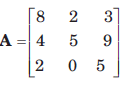is equal to:

Detailed Solution for Test: Linear Algebra - Question 3
• Since the sum of the eigenvalues of an n–square matrix is equal to the trace of the matrix (i.e. sum of the diagonal elements)
• So, required sum = 8 + 5 + 5  = 18
Test: Linear Algebra - Question 4

If 2, - 4 are the eigen values of a non–singular matrix A and |A| = 4, then the eigen values of adjA are:

Detailed Solution for Test: Linear Algebra - Question 4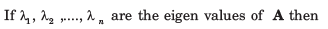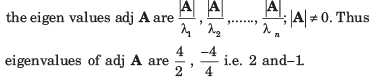Test: Linear Algebra - Question 5

If 2 and 4 are the eigen values of A then the eigenvalues of AT are

Detailed Solution for Test: Linear Algebra - Question 5

Since, the eigenvalues of A and Aare square so the eigenvalues of AT are 2 and 4.

Test: Linear Algebra - Question 6

If 1 and 3 are the eigenvalues of a square matrix A then A3 is equal to:

Detailed Solution for Test: Linear Algebra - Question 6
• Since 1 and 3 are the eigenvalues of A so the characteristic equation of A is: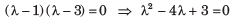• Also, by Cayley–Hamilton theorem, every square matrix satisfies its own characteristic equation. So: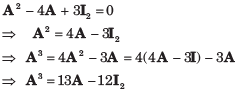Test: Linear Algebra - Question 7

If A is a square matrix of order 3 and |A| = 2 then A (adj A) is equal to:

Detailed Solution for Test: Linear Algebra - Question 7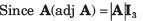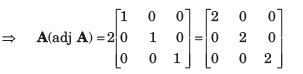Test: Linear Algebra - Question 8

If 1, 2 and 5 are the eigen values of the matrix A then |A| is equal to:

Detailed Solution for Test: Linear Algebra - Question 8

Since the product of the eigenvalues is equal to the determinant of the matrix so: |A| = 1 x 2 x 5 = 10

Test: Linear Algebra - Question 9

If the product of matrices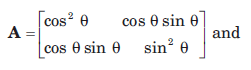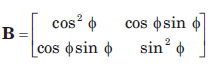is a null matrix, then θ and Ø differ by:

Detailed Solution for Test: Linear Algebra - Question 9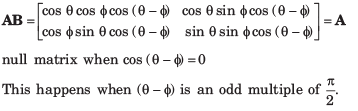Test: Linear Algebra - Question 10

If A and B are two matrices such that A +  B and AB are both defined, then A and B are:

Detailed Solution for Test: Linear Algebra - Question 10
• Since A + B is defined, A and B are matrices of the same type, say m x n. Also, AB is defined.
• So, the number of columns in A must be equal to the number of rows in B i.e. n = m.
• Hence, A and B are square matrices of the same order.
Test: Linear Algebra - Question 11

If A is a 3-rowed square matrix such that |A| = 2, then |adj(adj A2)| is equal to:

Detailed Solution for Test: Linear Algebra - Question 11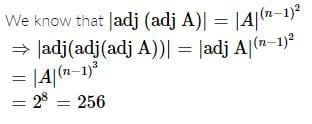Test: Linear Algebra - Question 12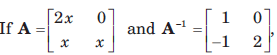then the value of x is:

Detailed Solution for Test: Linear Algebra - Question 12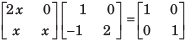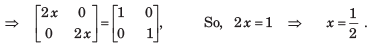Test: Linear Algebra - Question 13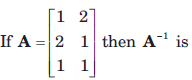Detailed Solution for Test: Linear Algebra - Question 13

Inverse matrix is defined for square matrix only.

Test: Linear Algebra - Question 14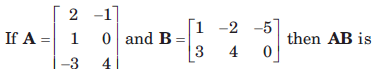Detailed Solution for Test: Linear Algebra - Question 14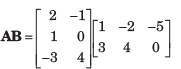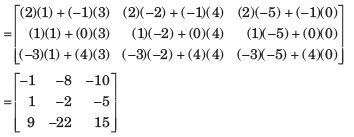Test: Linear Algebra - Question 15

A set of linear equations is represented by the matrix equation Ax = b. The necessary condition for the existence of a solution for this system is:

Detailed Solution for Test: Linear Algebra - Question 15

A must be invertible.

For a set of linear equations, Ax = b. The inverse of matrix A exists (i.e. |A| ≠ 0).

This is the necessary condition for the existence of a solution for this system.

Test: Linear Algebra - Question 16

Select a suitable figure from the four alternatives that would complete the figure matrix.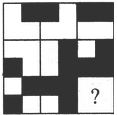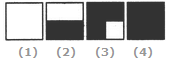Detailed Solution for Test: Linear Algebra - Question 16

In each row (as well as each column), the third figure is a combination of all the elements of the first and the second figures.

Test: Linear Algebra - Question 17

For a skew symmetric even ordered matrix A of integers, which of the following will not hold true:

Detailed Solution for Test: Linear Algebra - Question 17

Determinant of a skew-symmetric even ordered matrix A should be a perfect square.

Test: Linear Algebra - Question 18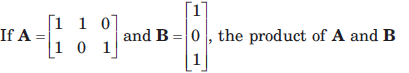Detailed Solution for Test: Linear Algebra - Question 18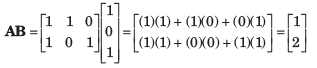Test: Linear Algebra - Question 19

Matrix D is an orthogonal matrix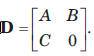The value of B is:

Detailed Solution for Test: Linear Algebra - Question 19

For orthogonal matrix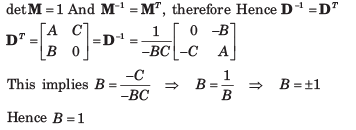Test: Linear Algebra - Question 20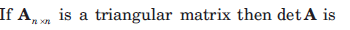Detailed Solution for Test: Linear Algebra - Question 20

From linear algebra for Anxn triangular matrix . DetA =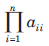The product of the diagonal entries of A.

## GATE Electrical Engineering (EE) 2023 Mock Test Series

22 docs|274 tests
 Use Code STAYHOME200 and get INR 200 additional OFF Use Coupon Code
Information about Test: Linear Algebra Page
In this test you can find the Exam questions for Test: Linear Algebra solved & explained in the simplest way possible. Besides giving Questions and answers for Test: Linear Algebra, EduRev gives you an ample number of Online tests for practice

## GATE Electrical Engineering (EE) 2023 Mock Test Series

22 docs|274 tests